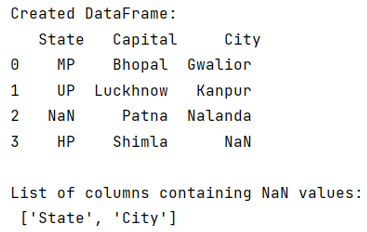# How to find which columns contain any NaN value in Pandas DataFrame?

Given a Pandas DataFrame, we have to find which columns contain any NaN value.
Submitted by Pranit Sharma, on June 01, 2022

While creating a DataFrame or importing a CSV file, there could be some NaN values in the cells. NaN values mean "Not a Number" which generally means that there are some missing values in the cell. To deal with this type of data, you can either remove the particular row (if the number of missing values is low) or you can handle these values.

To handle these values, we need to know which column contains NaN. For this purpose, we will first check if a column contains a NaN value or not by using the isna() method and then we will collect all the names of the column containing NaN values into a list by using the tolist() method.

To work with pandas, we need to import pandas package first, below is the syntax:

```import pandas as pd
```

Let us understand with the help of an example,

```# Importing pandas package
import pandas as pd

# Importing numpy package
import numpy as np

# Creating a Dictionary
d = {
'State':['MP','UP',np.NAN,'HP'],
'Capital':['Bhopal','Lucknow','Patna','Shimla'],
'City':['Gwalior','Kanpur','Nalanda',np.NAN]
}

# Creating a DataFrame
df = pd.DataFrame(d)

# Display DataFrame
print("Created DataFrame:\n",df,"\n")

# Checking which column contains NaN values
result = df.columns[df.isna().any()].tolist()

# Display result
print("List of columns containing NaN values:\n",result)
```

Output: